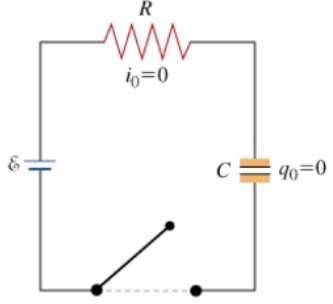# Problem: Consider a series circuit containing a resistor of resistance R and a capacitor of capacitance C connected to a source of EMF with negligible internal resistance. The wires are also assumed to have zero resistance. Initially, the switch is open and the capacitor discharged.Let us try to understand the processes that take place after the switch is closed. The charge of the capacitor, the current in the circuit, and, correspondingly, the voltages across the resistor and the capacitor will be changing. Note that at any moment in time during the life of our circuit, Kirchhoff's loop rule holds and, indeed it is helpful:ℰ - VR - VC = 0, where VR is the voltage across the resistor and VC is the voltage across the capacitorPart A.  Immediately after the switch is closed, what is the voltage across the capacitor? A) ℰB) 1/2 ℰC) zeroPart B.  Immediately after the switch is closed, what is the direction of the current in the circuit?A) clockwiseB) counterclockwiseC) There is no current because the capacitor does not allow the current to pass through.Part C.  Eventually, the process approaches a steady state. In that steady state, the charge of the capacitor is not changing. What is the current in the circuit in the steady state?A) ℰ/RB) ℰ/(R+C)C) ℰ/(R−C)D) ℰ/(C−R)E) zeroPart D.  In the steady state, what is the charge of q of the capacitor? Express your answer in terms of any or all of ℰ, R and C. Part E.  How much work W is done by the voltage source by the time the steady state is reached?

###### FREE Expert Solution

Part A

Charge, q = CV

V = q/C

Immediately after the switch is closed (at t=0), q = q0 = 0

79% (235 ratings)###### Problem Details

Consider a series circuit containing a resistor of resistance R and a capacitor of capacitance C connected to a source of EMF with negligible internal resistance. The wires are also assumed to have zero resistance. Initially, the switch is open and the capacitor discharged.

Let us try to understand the processes that take place after the switch is closed. The charge of the capacitor, the current in the circuit, and, correspondingly, the voltages across the resistor and the capacitor will be changing. Note that at any moment in time during the life of our circuit, Kirchhoff's loop rule holds and, indeed it is helpful:

ℰ - VR - VC = 0, where VR is the voltage across the resistor and VC is the voltage across the capacitorPart A.  Immediately after the switch is closed, what is the voltage across the capacitor?

A) ℰ
B) 1/2 ℰ
C) zero

Part B.  Immediately after the switch is closed, what is the direction of the current in the circuit?

A) clockwise
B) counterclockwise
C) There is no current because the capacitor does not allow the current to pass through.

Part C.  Eventually, the process approaches a steady state. In that steady state, the charge of the capacitor is not changing. What is the current in the circuit in the steady state?

A) ℰ/R
B) ℰ/(R+C)
C) ℰ/(R−C)
D) ℰ/(C−R)
E) zero

Part D.  In the steady state, what is the charge of q of the capacitor?

Express your answer in terms of any or all of ℰ, R and C.

Part E.  How much work W is done by the voltage source by the time the steady state is reached?